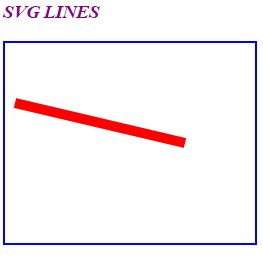# Lines in HTML5

### Lines

We can create the lines in SVG using HTML5

The syntax for creating lines is

```    x1 - It is the starting point of the x axis
y1 - It is the starting point of the y axis
x2 - It is the ending point of the x axis
y2 - It id the ending point of the y axis
```

### Creating Lines

We can create the lines in SVG using HTML5

```<h3 style="color:darkmagenta"><i>SVG LINES</i></h3>
<svg width="250" height="200" style="border:2px solid blue">
<line x1="10" y1="60" x2="180" y2="100"
stroke="red" stroke-width="10"></line>
</svg>
```

Lines

In the above code snippet we have defined the lines using svg

• x1 - It is the starting point of the x axis
• y1 - It is the starting point of the y axis
• x2 - It is the ending point of the x axis
• y2 - It id the ending point of the y axis
• stroke() - It defines the stroke color as red
• stroke width() - It defines the width of the line as 10

outputViews: 2041 | Post Order: 123